Скачать презентацию Solving Inequalities by 3 -2 Adding or Subtracting

b7c5af884fdb22391d8e20c48bb1955a.ppt

• Количество слайдов: 33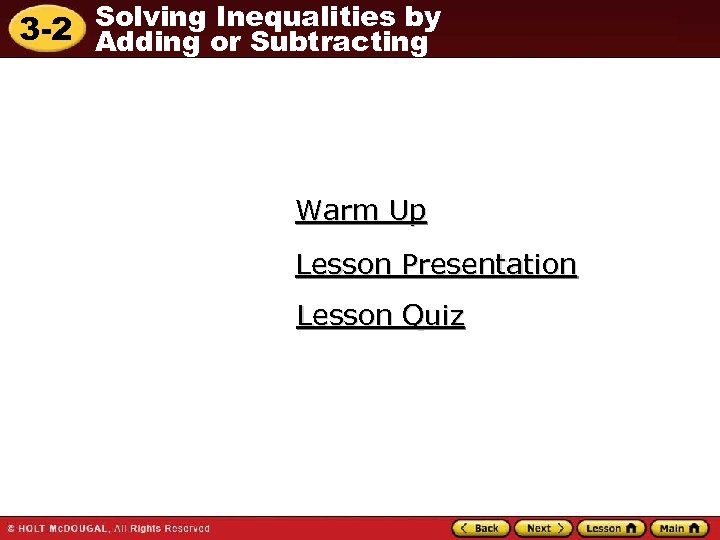Solving Inequalities by 3 -2 Adding or Subtracting Warm Up Lesson Presentation Lesson Quiz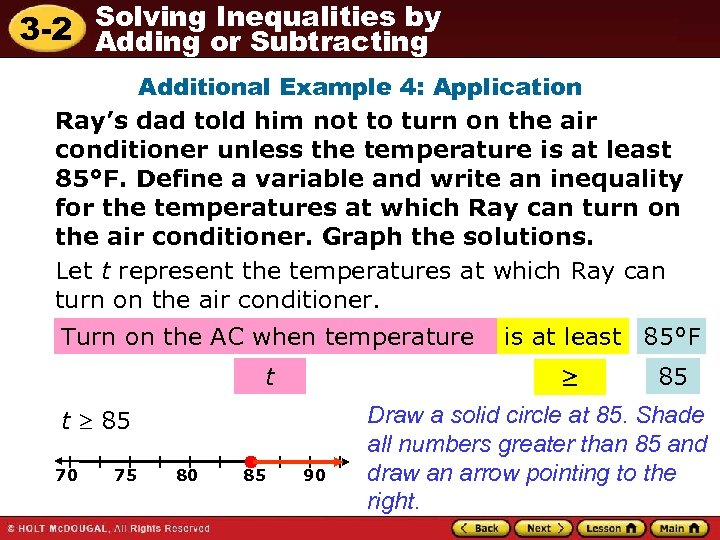Solving Inequalities by 3 -2 Adding or Subtracting Additional Example 4: Application Ray’s dad told him not to turn on the air conditioner unless the temperature is at least 85°F. Define a variable and write an inequality for the temperatures at which Ray can turn on the air conditioner. Graph the solutions. Let t represent the temperatures at which Ray can turn on the air conditioner. Turn on the AC when temperature t ≥ t 85 70 75 80 85 is at least 85°F 90 85 Draw a solid circle at 85. Shade all numbers greater than 85 and draw an arrow pointing to the right.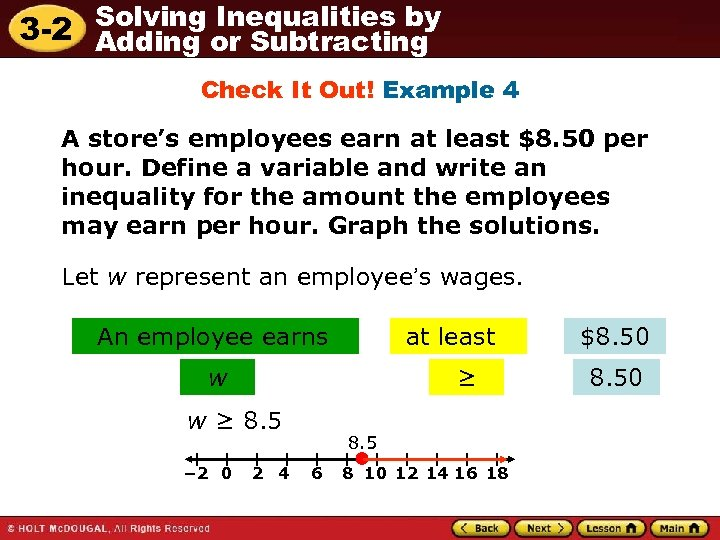Solving Inequalities by 3 -2 Adding or Subtracting Check It Out! Example 4 A store’s employees earn at least \$8. 50 per hour. Define a variable and write an inequality for the amount the employees may earn per hour. Graph the solutions. Let w represent an employee’s wages. An employee earns at least w ≥ 8. 5 − 2 0 2 4 8. 5 6 8 10 12 14 16 18 \$8. 50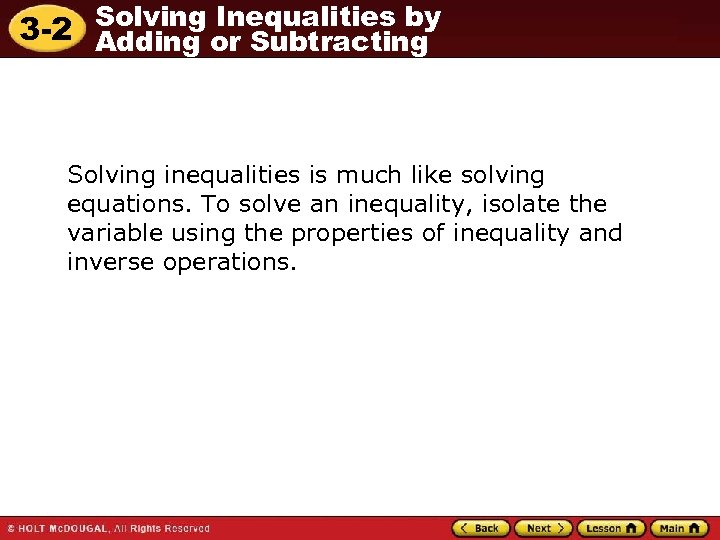Solving Inequalities by 3 -2 Adding or Subtracting Solving inequalities is much like solving equations. To solve an inequality, isolate the variable using the properties of inequality and inverse operations.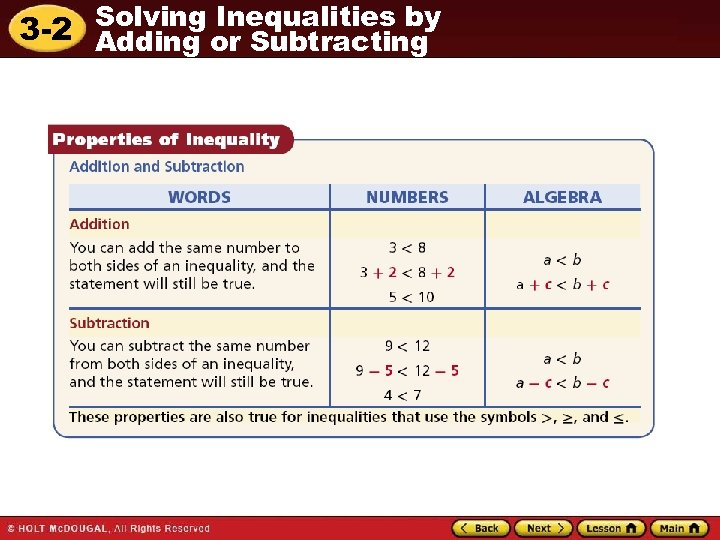Solving Inequalities by 3 -2 Adding or Subtracting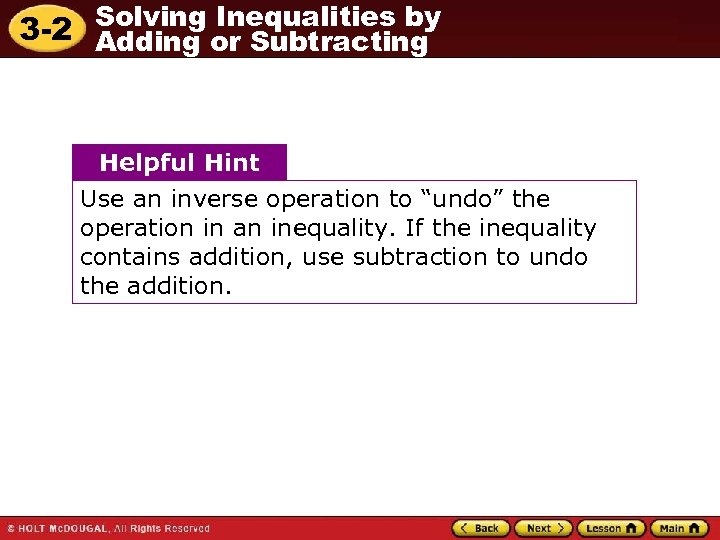Solving Inequalities by 3 -2 Adding or Subtracting Helpful Hint Use an inverse operation to “undo” the operation in an inequality. If the inequality contains addition, use subtraction to undo the addition.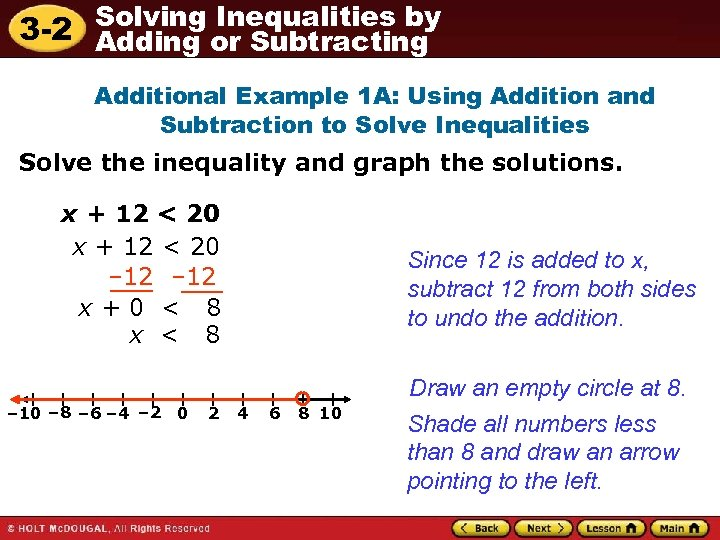Solving Inequalities by 3 -2 Adding or Subtracting Additional Example 1 A: Using Addition and Subtraction to Solve Inequalities Solve the inequality and graph the solutions. x + 12 < 20 – 12 x+0 < 8 x < 8 – 10 – 8 – 6 – 4 – 2 0 2 Since 12 is added to x, subtract 12 from both sides to undo the addition. 4 6 8 10 Draw an empty circle at 8. Shade all numbers less than 8 and draw an arrow pointing to the left.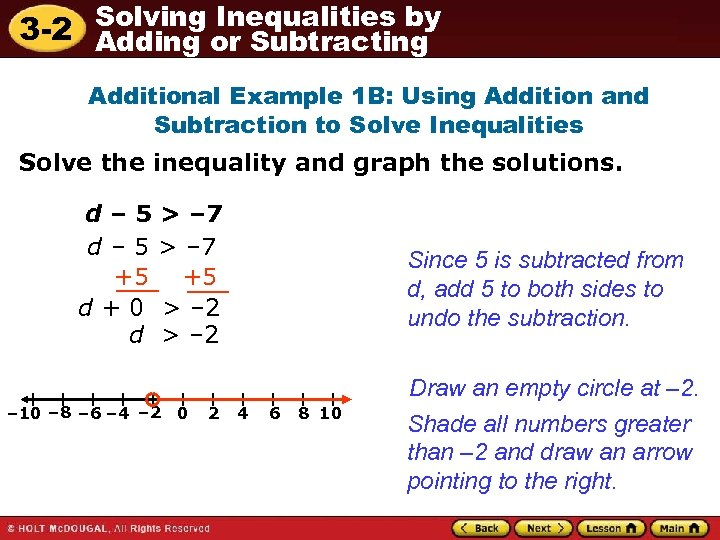Solving Inequalities by 3 -2 Adding or Subtracting Additional Example 1 B: Using Addition and Subtraction to Solve Inequalities Solve the inequality and graph the solutions. d – 5 > – 7 +5 +5 d + 0 > – 2 d > – 2 – 10 – 8 – 6 – 4 – 2 0 2 Since 5 is subtracted from d, add 5 to both sides to undo the subtraction. 4 6 8 10 Draw an empty circle at – 2. Shade all numbers greater than – 2 and draw an arrow pointing to the right.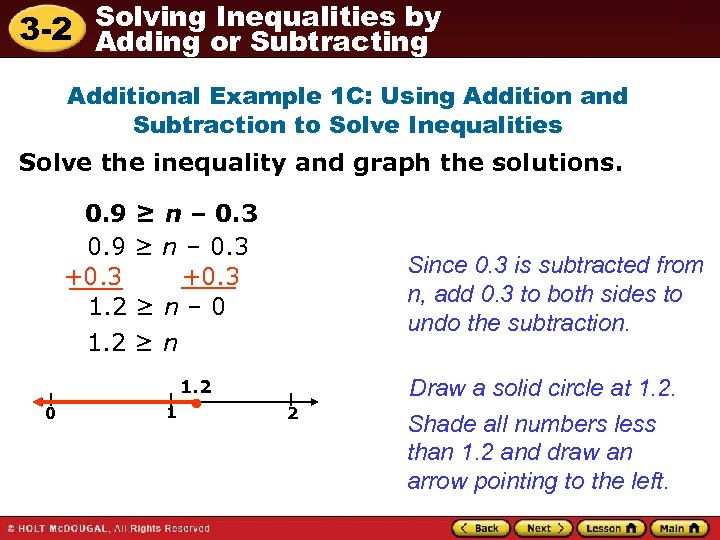Solving Inequalities by 3 -2 Adding or Subtracting Additional Example 1 C: Using Addition and Subtraction to Solve Inequalities Solve the inequality and graph the solutions. 0. 9 ≥ n – 0. 3 +0. 3 1. 2 ≥ n – 0 1. 2 ≥ n Since 0. 3 is subtracted from n, add 0. 3 to both sides to undo the subtraction. 1. 2 0 1 2 Draw a solid circle at 1. 2. Shade all numbers less than 1. 2 and draw an arrow pointing to the left.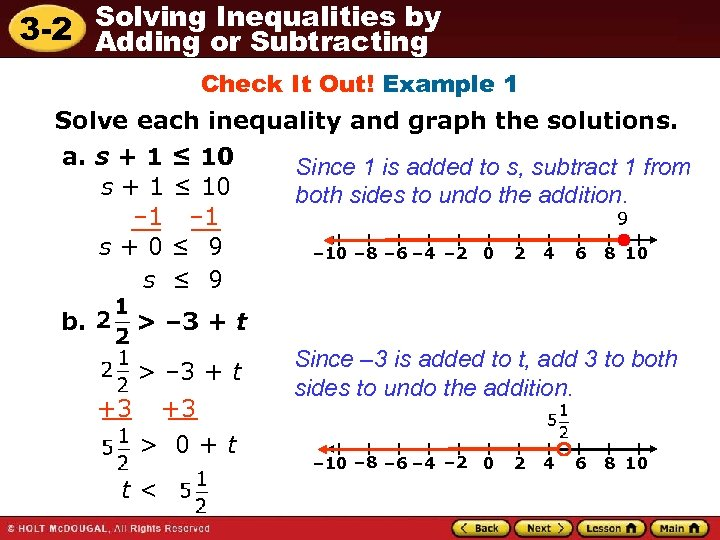Solving Inequalities by 3 -2 Adding or Subtracting Check It Out! Example 1 Solve each inequality and graph the solutions. a. s + 1 ≤ 10 – 1 s+0≤ 9 s ≤ 9 b. Since 1 is added to s, subtract 1 from both sides to undo the addition. 9 – 10 – 8 – 6 – 4 – 2 0 2 4 6 8 10 > – 3 + t +3 +3 > 0+t t< Since – 3 is added to t, add 3 to both sides to undo the addition. – 10 – 8 – 6 – 4 – 2 0 2 4 6 8 10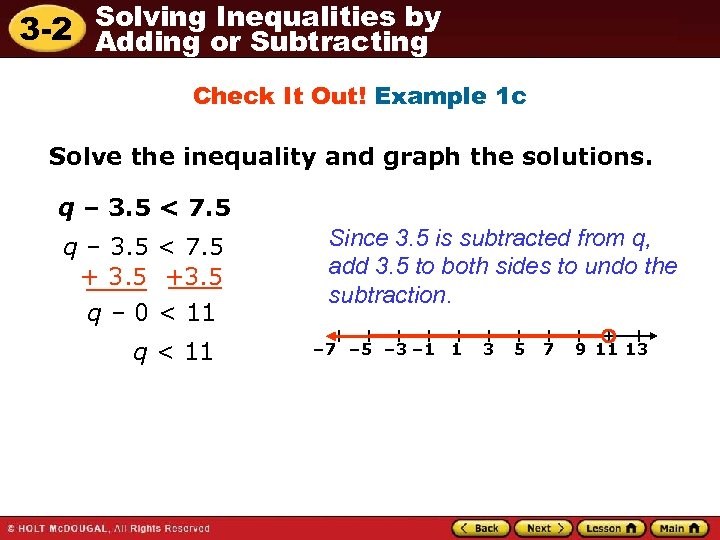Solving Inequalities by 3 -2 Adding or Subtracting Check It Out! Example 1 c Solve the inequality and graph the solutions. q – 3. 5 < 7. 5 + 3. 5 +3. 5 q – 0 < 11 q < 11 Since 3. 5 is subtracted from q, add 3. 5 to both sides to undo the subtraction. – 7 – 5 – 3 – 1 1 3 5 7 9 11 13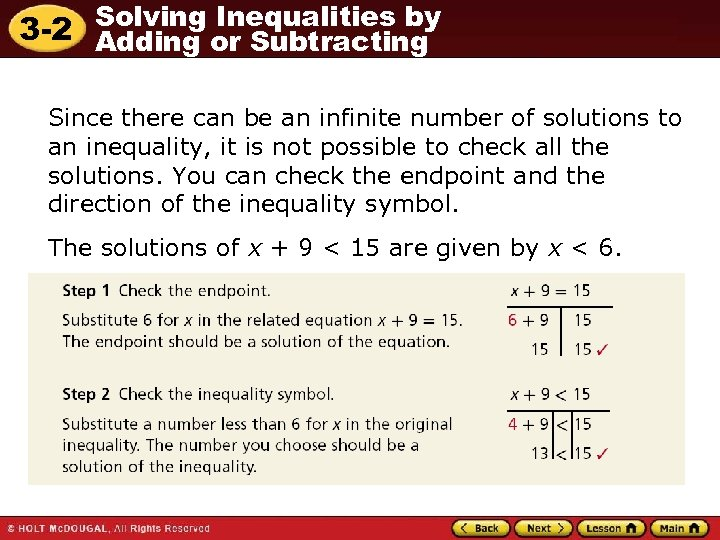Solving Inequalities by 3 -2 Adding or Subtracting Since there can be an infinite number of solutions to an inequality, it is not possible to check all the solutions. You can check the endpoint and the direction of the inequality symbol. The solutions of x + 9 < 15 are given by x < 6.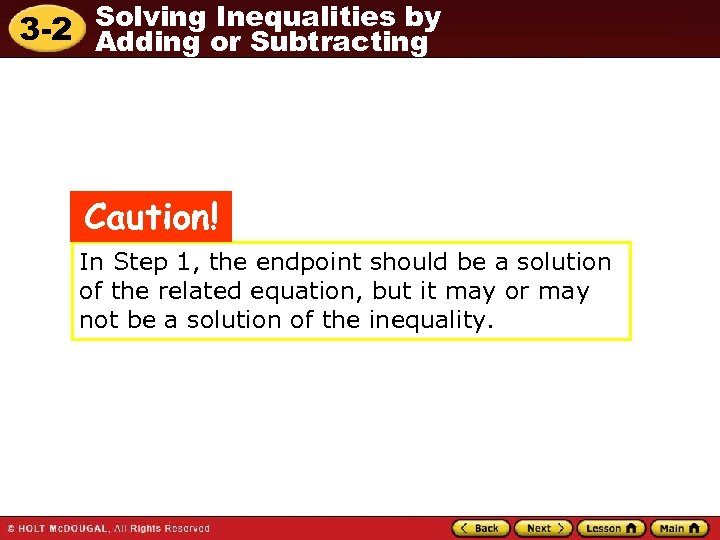Solving Inequalities by 3 -2 Adding or Subtracting Caution! In Step 1, the endpoint should be a solution of the related equation, but it may or may not be a solution of the inequality.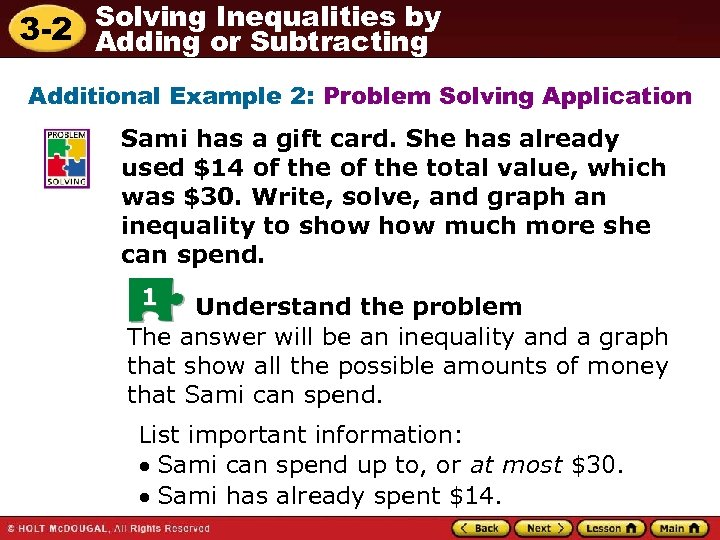Solving Inequalities by 3 -2 Adding or Subtracting Additional Example 2: Problem Solving Application Sami has a gift card. She has already used \$14 of the total value, which was \$30. Write, solve, and graph an inequality to show much more she can spend. 1 Understand the problem The answer will be an inequality and a graph that show all the possible amounts of money that Sami can spend. List important information: • Sami can spend up to, or at most \$30. • Sami has already spent \$14.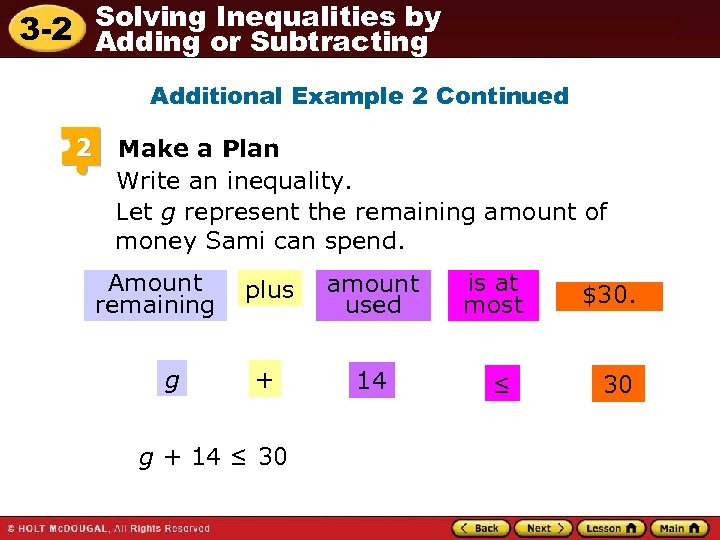Solving Inequalities by 3 -2 Adding or Subtracting Additional Example 2 Continued 2 Make a Plan Write an inequality. Let g represent the remaining amount of money Sami can spend. Amount remaining g plus amount used + 14 g + 14 ≤ 30 is at most ≤ \$30. 30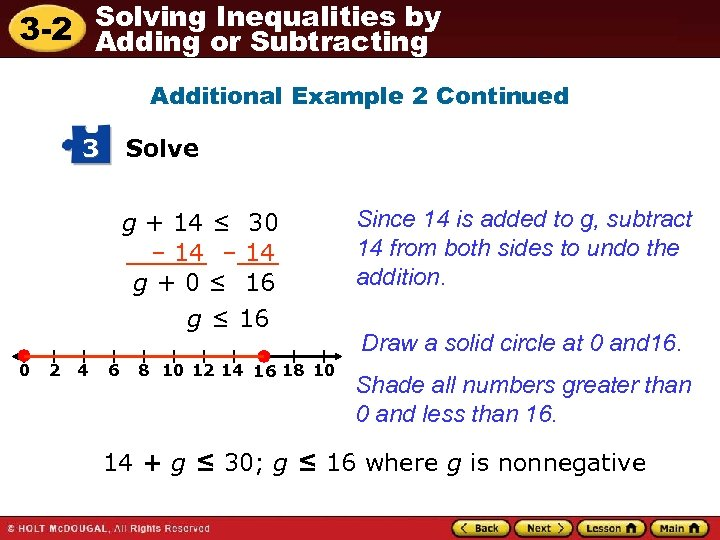Solving Inequalities by 3 -2 Adding or Subtracting Additional Example 2 Continued 3 Solve g + 14 ≤ 30 – 14 g + 0 ≤ 16 g ≤ 16 0 2 4 6 8 10 12 14 16 18 10 Since 14 is added to g, subtract 14 from both sides to undo the addition. Draw a solid circle at 0 and 16. Shade all numbers greater than 0 and less than 16. 14 + g ≤ 30; g ≤ 16 where g is nonnegative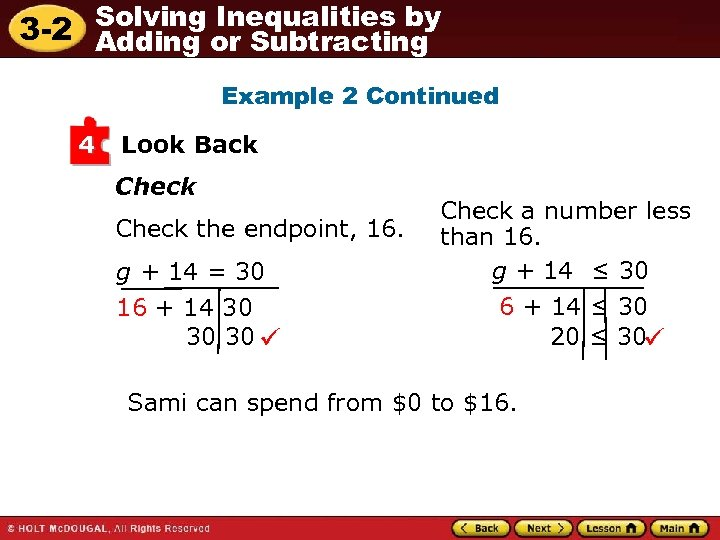Solving Inequalities by 3 -2 Adding or Subtracting Example 2 Continued 4 Look Back Check the endpoint, 16. g + 14 = 30 16 + 14 30 30 30 Check a number less than 16. g + 14 ≤ 30 6 + 14 ≤ 30 20 ≤ 30 Sami can spend from \$0 to \$16.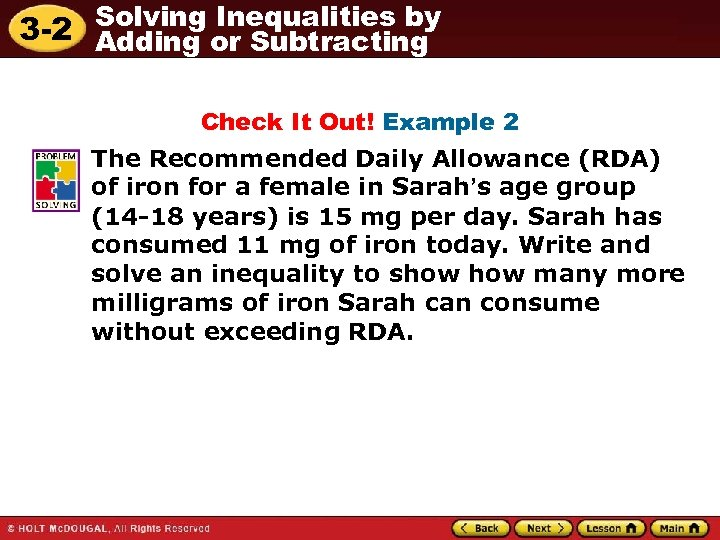Solving Inequalities by 3 -2 Adding or Subtracting Check It Out! Example 2 The Recommended Daily Allowance (RDA) of iron for a female in Sarah’s age group (14 -18 years) is 15 mg per day. Sarah has consumed 11 mg of iron today. Write and solve an inequality to show many more milligrams of iron Sarah can consume without exceeding RDA.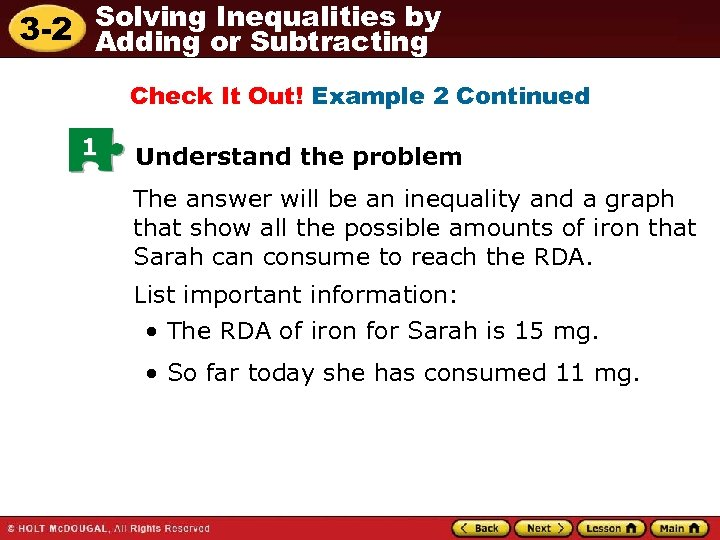Solving Inequalities by 3 -2 Adding or Subtracting Check It Out! Example 2 Continued 1 Understand the problem The answer will be an inequality and a graph that show all the possible amounts of iron that Sarah can consume to reach the RDA. List important information: • The RDA of iron for Sarah is 15 mg. • So far today she has consumed 11 mg.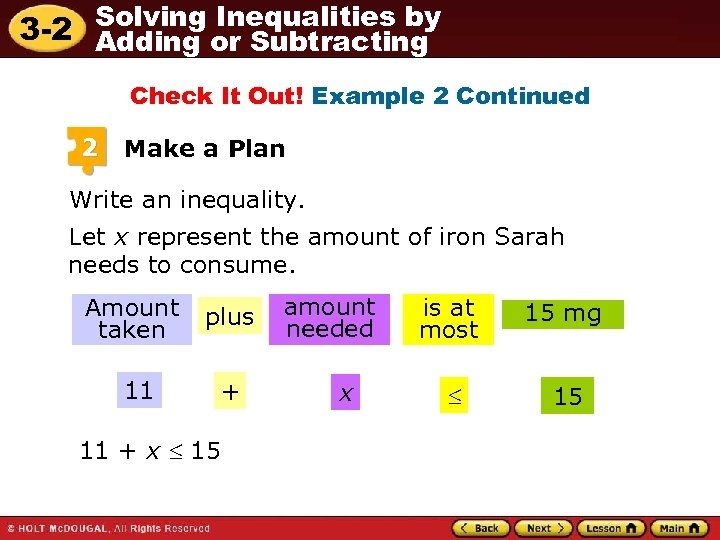Solving Inequalities by 3 -2 Adding or Subtracting Check It Out! Example 2 Continued 2 Make a Plan Write an inequality. Let x represent the amount of iron Sarah needs to consume. Amount taken 11 plus + 11 + x 15 amount needed x is at most 15 mg 15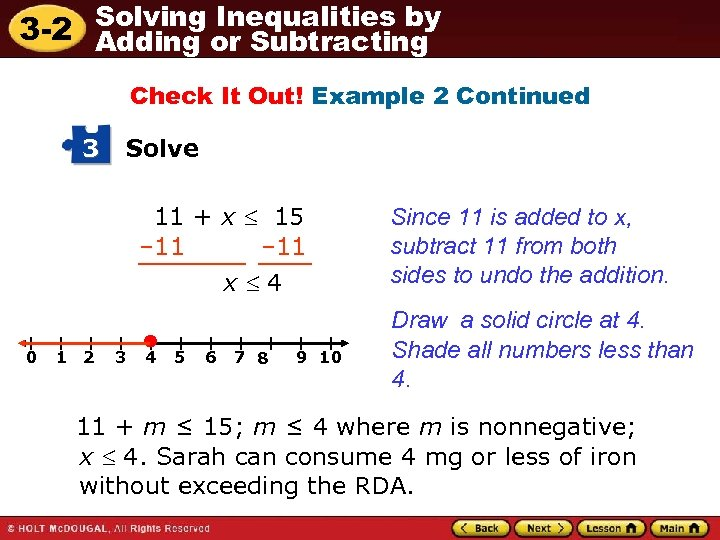Solving Inequalities by 3 -2 Adding or Subtracting Check It Out! Example 2 Continued 3 Solve 11 + x 15 – 11 x 4 0 1 2 3 4 5 6 7 8 9 10 Since 11 is added to x, subtract 11 from both sides to undo the addition. Draw a solid circle at 4. Shade all numbers less than 4. 11 + m ≤ 15; m ≤ 4 where m is nonnegative; x 4. Sarah can consume 4 mg or less of iron without exceeding the RDA.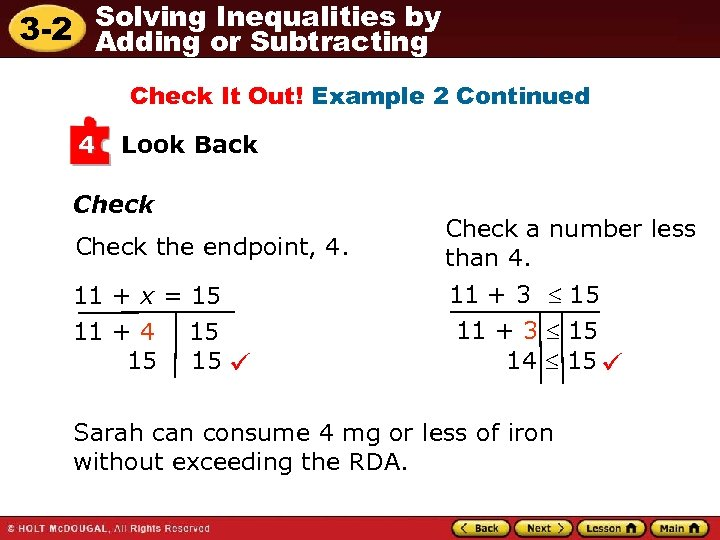Solving Inequalities by 3 -2 Adding or Subtracting Check It Out! Example 2 Continued 4 Look Back Check the endpoint, 4. Check a number less than 4. 11 + x = 15 11 + 4 15 15 15 11 + 3 15 14 15 Sarah can consume 4 mg or less of iron without exceeding the RDA.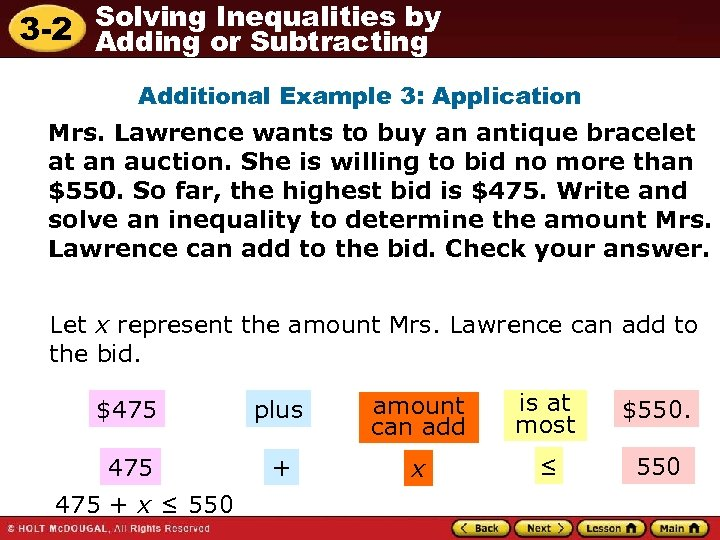Solving Inequalities by 3 -2 Adding or Subtracting Additional Example 3: Application Mrs. Lawrence wants to buy an antique bracelet at an auction. She is willing to bid no more than \$550. So far, the highest bid is \$475. Write and solve an inequality to determine the amount Mrs. Lawrence can add to the bid. Check your answer. Let x represent the amount Mrs. Lawrence can add to the bid. \$475 plus amount can add is at most \$550. 475 + x ≤ 550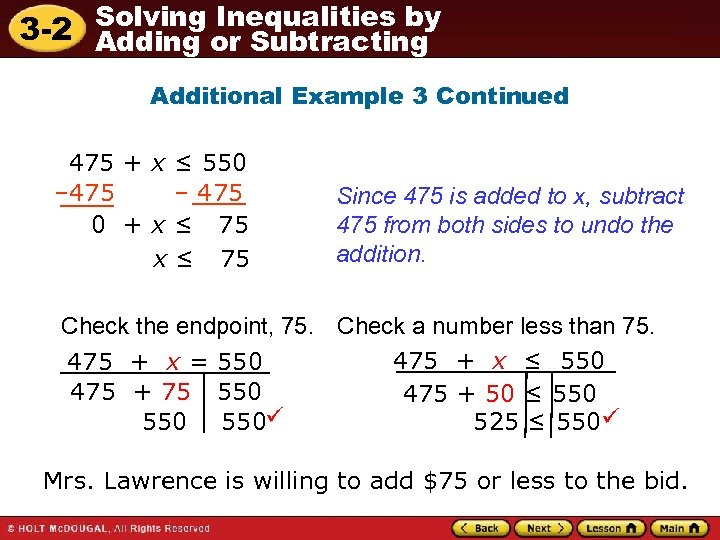Solving Inequalities by 3 -2 Adding or Subtracting Additional Example 3 Continued 475 + x ≤ 550 – 475 0 + x ≤ 75 Since 475 is added to x, subtract 475 from both sides to undo the addition. Check the endpoint, 75. Check a number less than 75. 475 + x ≤ 550 475 + x = 550 475 + 75 550 475 + 50 ≤ 550 525 ≤ 550 550 Mrs. Lawrence is willing to add \$75 or less to the bid.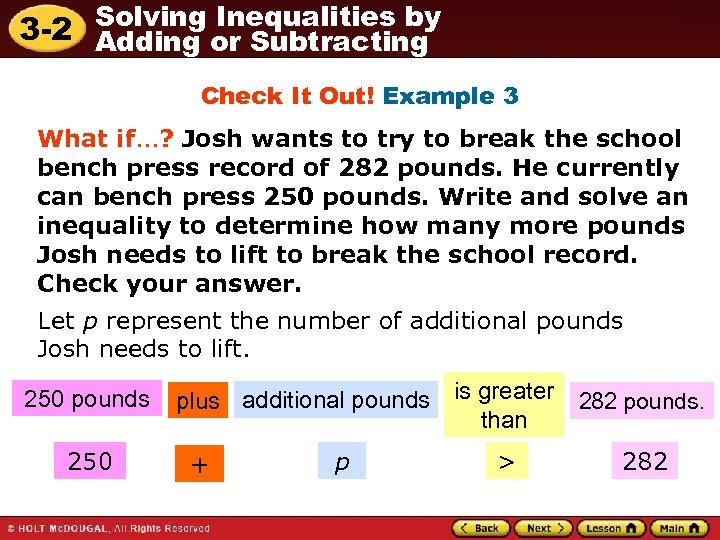Solving Inequalities by 3 -2 Adding or Subtracting Check It Out! Example 3 What if…? Josh wants to try to break the school bench press record of 282 pounds. He currently can bench press 250 pounds. Write and solve an inequality to determine how many more pounds Josh needs to lift to break the school record. Check your answer. Let p represent the number of additional pounds Josh needs to lift. 250 pounds 250 plus additional pounds is greater than + p > 282 pounds. 282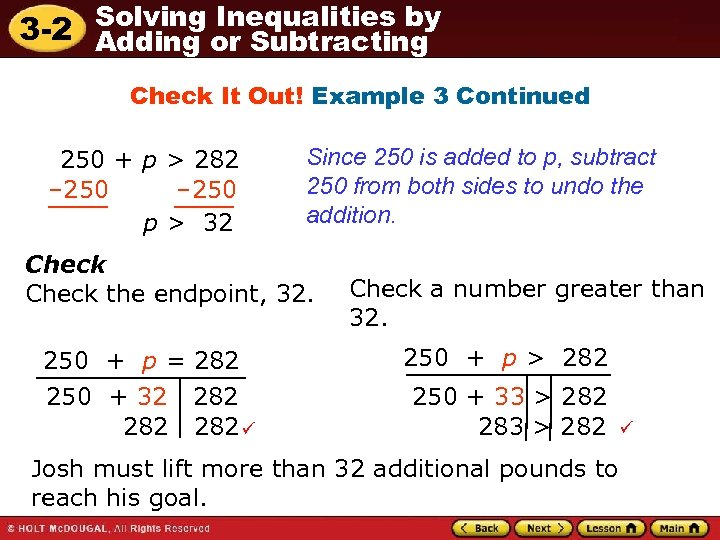Solving Inequalities by 3 -2 Adding or Subtracting Check It Out! Example 3 Continued 250 + p > 282 – 250 p > 32 Since 250 is added to p, subtract 250 from both sides to undo the addition. Check the endpoint, 32. 250 + p = 282 250 + 32 282 282 Check a number greater than 32. 250 + p > 282 250 + 33 > 282 283 > 282 Josh must lift more than 32 additional pounds to reach his goal.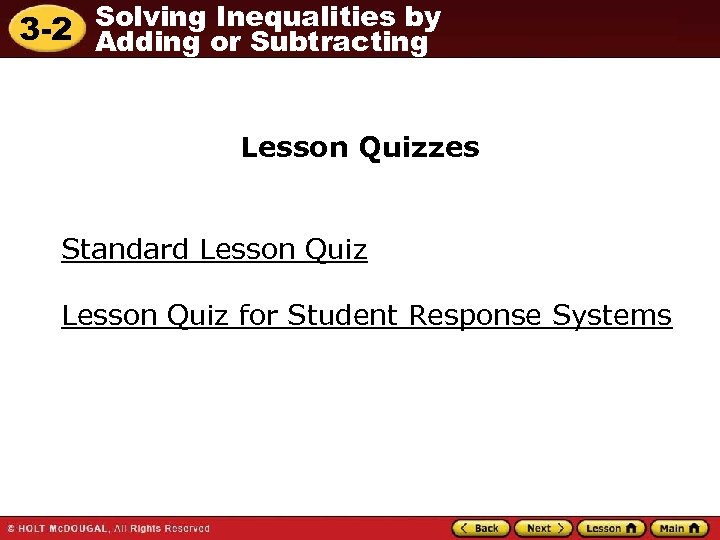Solving Inequalities by 3 -2 Adding or Subtracting Lesson Quizzes Standard Lesson Quiz for Student Response Systems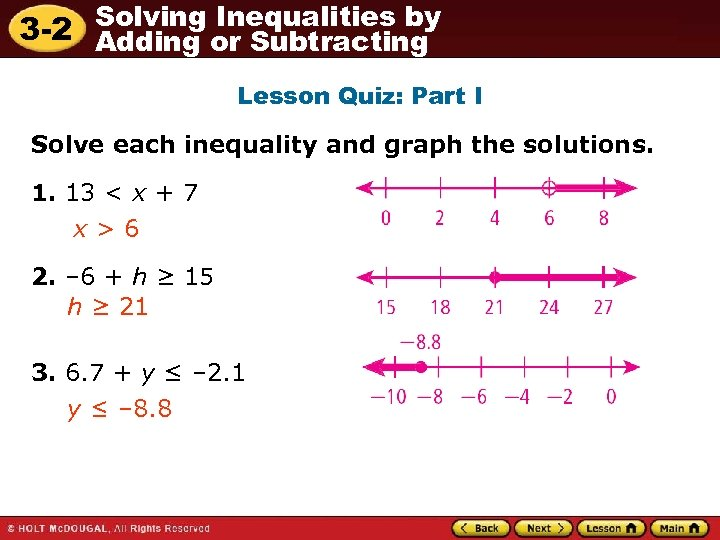Solving Inequalities by 3 -2 Adding or Subtracting Lesson Quiz: Part I Solve each inequality and graph the solutions. 1. 13 < x + 7 x>6 2. – 6 + h ≥ 15 h ≥ 21 3. 6. 7 + y ≤ – 2. 1 y ≤ – 8. 8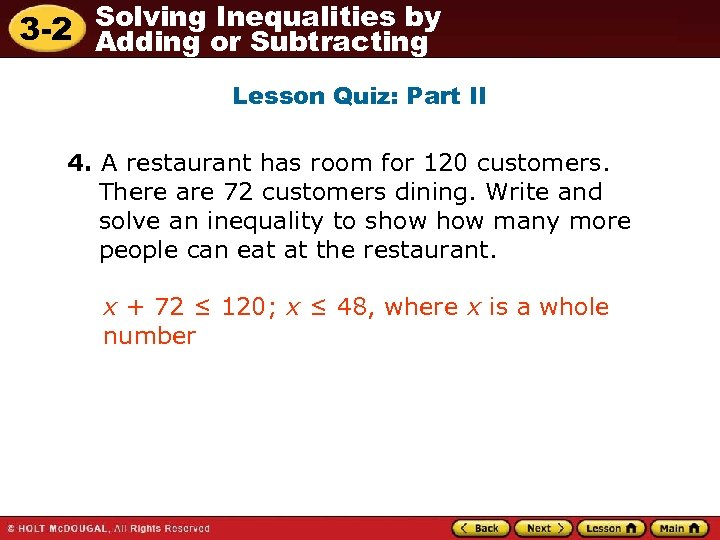Solving Inequalities by 3 -2 Adding or Subtracting Lesson Quiz: Part II 4. A restaurant has room for 120 customers. There are 72 customers dining. Write and solve an inequality to show many more people can eat at the restaurant. x + 72 ≤ 120; x ≤ 48, where x is a whole number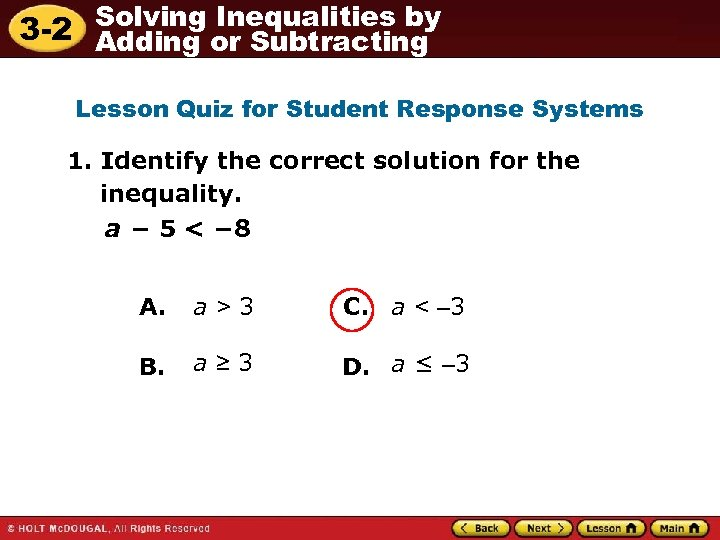Solving Inequalities by 3 -2 Adding or Subtracting Lesson Quiz for Student Response Systems 1. Identify the correct solution for the inequality. a − 5 < − 8 A. a>3 C. a < – 3 B. a≥ 3 D. a ≤ – 3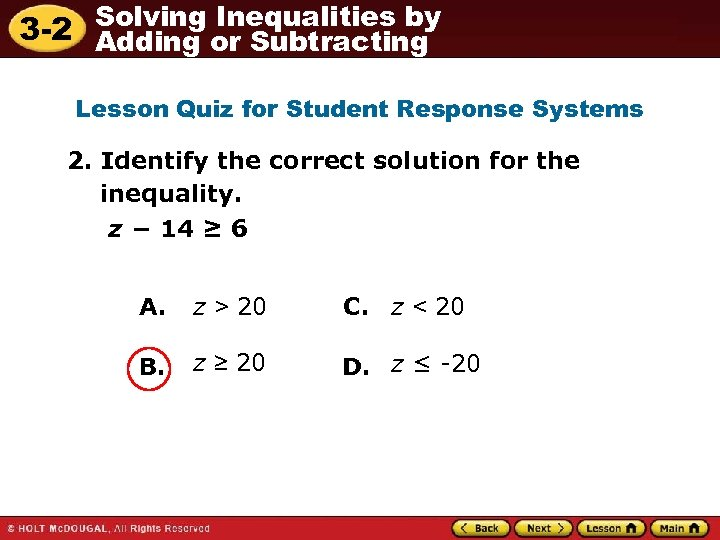Solving Inequalities by 3 -2 Adding or Subtracting Lesson Quiz for Student Response Systems 2. Identify the correct solution for the inequality. z − 14 ≥ 6 A. z > 20 C. z < 20 B. z ≥ 20 D. z ≤ -20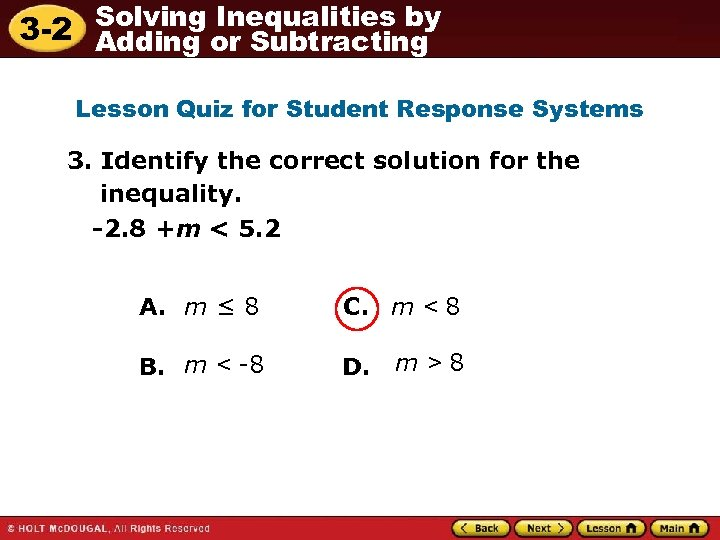Solving Inequalities by 3 -2 Adding or Subtracting Lesson Quiz for Student Response Systems 3. Identify the correct solution for the inequality. -2. 8 +m < 5. 2 A. m ≤ 8 C. m < 8 B. m < -8 D. m>8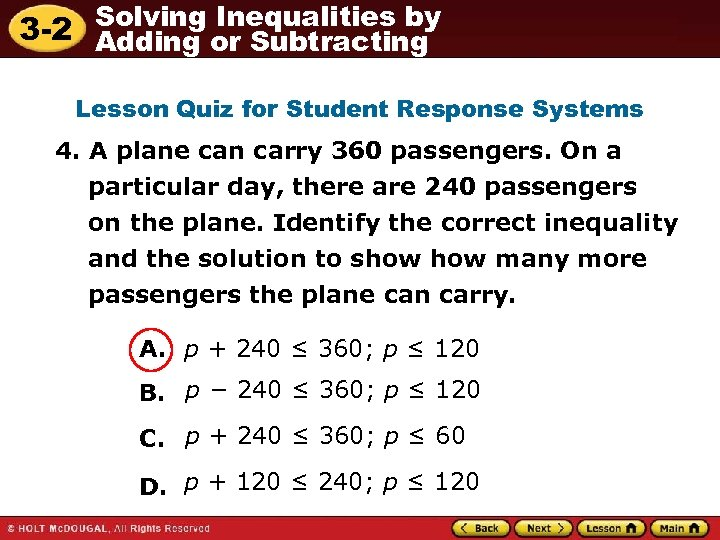Solving Inequalities by 3 -2 Adding or Subtracting Lesson Quiz for Student Response Systems 4. A plane can carry 360 passengers. On a particular day, there are 240 passengers on the plane. Identify the correct inequality and the solution to show many more passengers the plane can carry. A. p + 240 ≤ 360; p ≤ 120 B. p − 240 ≤ 360; p ≤ 120 C. p + 240 ≤ 360; p ≤ 60 D. p + 120 ≤ 240; p ≤ 120